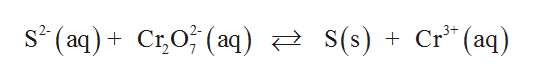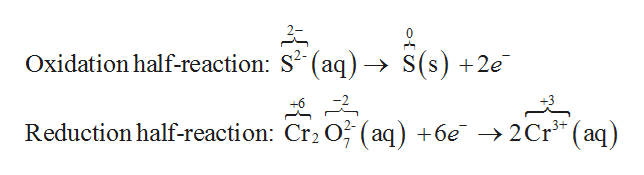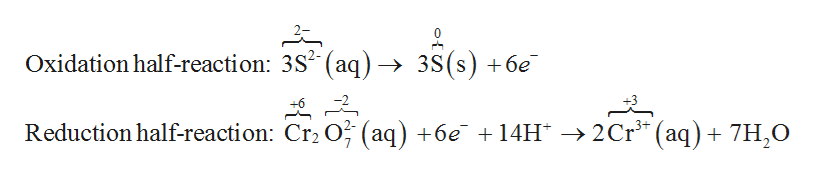# The following change occurs in acidic solution:____ S2–(aq) + ____ Cr2O72–(aq) → ____ S(s) + ____ Cr3+(aq)In the balanced equation, for every mole of Cr2O72– that reacts, _____ mol of H+ are consumed.a. 7b. 14c. 5d. 10e. 8

Question
2 views

The following change occurs in acidic solution:

____ S2–(aq) + ____ Cr2O72–(aq) → ____ S(s) + ____ Cr3+(aq)

In the balanced equation, for every mole of Cr2O72– that reacts, _____ mol of H+ are consumed.

a. 7

b. 14

c. 5

d. 10

e. 8

check_circle

Step 1

The given reaction is,help_outlineImage Transcriptioncloses* (aq) + C;O; (aq) स्टे S( s ) + Cr " (aq) C;O; (aq) स्टे s(s ) fullscreen
Step 2

Step (1): separate the above reaction into two half reactions and balance all other atoms except hydrogen and Oxygen.help_outlineImage TranscriptioncloseOxidation half-reaction: S (aq) S(s) +2e Reduction half-reaction: Cr2 O (aq) +6e -2 Cr3* (aq) fullscreen
Step 3

Step (2): Balance the charge by adding H+ ion and balance the Oxygen by add...help_outlineImage TranscriptioncloseOxidation half-reaction: 3S2 (aq) -» 3S(s) +6e Reduction half-reaction: Cr2 O (aq) +6e 14H ->2Cr (aq) 7H,O fullscreen

### Want to see the full answer?

See Solution

#### Want to see this answer and more?

Solutions are written by subject experts who are available 24/7. Questions are typically answered within 1 hour.*

See Solution
*Response times may vary by subject and question.
Tagged in

### General Chemistry